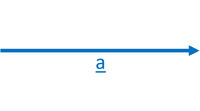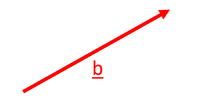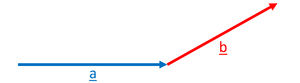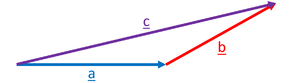# Vector

## Key Stage 4

### Meaning

A vector is a quantity with both magnitude and direction.

Vector quantities include:

• Forces \(\overrightarrow{F}\) - A push or a pull which has a magnitude (size) and a direction.
• Displacement \(\overrightarrow{s}\) - The distance and direction from one point in space to another.
• Velocity \(\overrightarrow{v}\) - The speed and direction that an object is moving.
• Acceleration \(\overrightarrow{a}\) - The rate of change of velocity and the direction of this change.

If a quantity does not include direction, then it is called a scalar, not a vector.

### Representing Vectors

Vectors are written with either an underline (\(\underline{F}\),\(\underline{s}\),\(\underline{v}\),\(\underline{a}\)) or an arrow above (\(\overrightarrow{F}\),\(\overrightarrow{s}\),\(\overrightarrow{v}\),\(\overrightarrow{a}\)).
Vectors are often drawn on diagrams. They are drawn as an arrow because an arrow can show the magnitude (the length of the arrow) and the direction (which way the arrow is pointing).The vector \(\underline{a}\) is shown by this arrow. The length of the arrow represents the magnitude of the vector and the direction of the arrow shows the direction of the vector. The vector \(\underline{b}\) is shown by this arrow. The length of the arrow represents the magnitude of the vector and the direction of the arrow shows the direction of the vector. The arrow \(\underline{b}\) is shorter than the arrow \(\underline{a}\) so the vector \(\underline{b}\) has a smaller magnitude than \(\underline{a}\). It can also be seen that vector \(\underline{b}\) has a different direction to vector \(\underline{a}\).
Using a diagram two vector quantities can be added together by drawing the arrows end to end.The two vectors can be added by placing the start of one vector at the end of the other. A new vector \(\underline{c}\) is created from the addition of \(\underline{a}\) and \(\underline{b}\). \(\underline{a}+\underline{b}=\underline{c}\) If the vectors are drawn accurately in length using a ruler and in direction using a protractor the length and direction of the new vector can be measured from the diagram. This type of Scale Diagram can be used to find the magnitude and direction of the new vector without calculation.